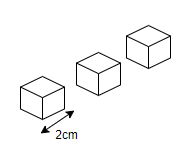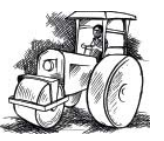# Practice questions for Mensurations

In this page we have Practice questions for Mensurations Class 8 maths Chapter 11 . Hope you like them and do not forget to like , social share and comment at the end of the page.
Question 1
There are two cuboidal whose dimensions are given below. Which box requires the higher amount of material to make?
Cuboid A: L=23, B=30, H=40
Cuboid B: L=30, B=12, H=44

Question 2
Three cubes, each of edge 2 cm. long are placed together. Find the total surface area of the cuboid so formed?Question 3
Find the side of a cube whose surface area is 2400 cm2.

Question 4
Meghna painted the outside of the cabinet of measure 2 m × 3 m × 2.5 m. How much surface area did she cover if she painted all except the bottom of the cabinet and back side?

Question 5
Ahmed is painting the walls and ceiling of a cuboidal hall with length, breadth and height of 25 m, 12 m and 8 m respectively. From each can of paint 200 m² of area is painted. How many cans of paint will she need to paint the room?

Question 6
A open cylindrical tank of radius 14 m and height 3 m is made from a sheet of metal. How much sheet of metal is required?

Question 7
The lateral surface area of a hollow cylinder is 4224 cm2. It is cut along its height and formed a rectangular sheet of width 33 cm. Find the perimeter of rectangular sheet?

Question 8
A road roller takes 750 complete revolutions to move once over to level a road. Find the area of the road if the diameter of a road roller is 154 cm and length is 1 m.Question 9
A rectangular sheet of metal foil is 88 cm. long and 20 cm. wide. A cylinder is made out of it, by rolling the foil along width. Find the volume of the cylinder.

Question 10
The perimeter of the floor of a hall is 250 m. If the height is 4 m, find the cost of painting the four walls at the rate of Rs. 12 per square meter.

Question 12
How many times do the volume and surface area of a cube increase if its edges get tripled.

Question 13
How many times do the volume and surface area of a cylinder increase if its radius doubled and height remains same

Question 14
How many times do the volume and surface area of a cylinder increase if its radius remains same and height is doubled

Question 15
The height of a cylinder is 15 cm. and curved surface area is 660 cm2. Find the radius of the cylinder

## Summary

This Practice questions for Mensurations Class 8 maths is prepared keeping in mind the latest syllabus of CBSE . This has been designed in a way to improve the academic performance of the students. If you find mistakes , please do provide the feedback on the mail.